top of page

# Using RTA to Aid ResFrac History Matching

Introduction and Motivation

Rate Transient Analysis (RTA) is commonly used to analyze production in unconventional reservoirs. The concept of RTA is to use rate signatures of producing wells to estimate properties such as permeability and fracture surface area. For more detailed analysis, fracture simulators, reservoir simulators, and coupled fracture/reservoir simulators, like ResFrac, can be used.

At ResFrac, we use RTA and ResFrac together to expedite the history matching process by gaining intuition into which variables to adjust and by what degree. There are specific motifs seen in RTA trends that give insight into the processes at play. By comparing RTA trends from measured data and simulated data, we can identify mechanisms that may be responsible for the trends observed in the measured data. Combining with knowledge of the play and heuristic data, we can eliminate or constrain the list of possible mechanisms.

The overall ResFrac modeling process is:

1. Set up a ResFrac model with geologic properties, wellbore and completion designs, and fracturing and production schedules

2. Run the initial model

3. Use microseismic, frac hit data, DAS, and other data to tune the fracture geometries

4. Plot RTA trends of the real and simulated data

5. Use motifs presented below to identify possible production mechanisms in the real data

6. Adjust history matching parameters in the ResFrac simulation based on the intuition gained from RTA of the real data

7. Iterate steps 4-6 to obtain a match in RTA trends

8. Depending on reservoir type, some additional tweaking of relative permeabilities may be required to get correct production of oil, water, and gas

In the remainder of this blog post I will cover the methods used to plot RTA trends and several RTA motifs that can be used to identify specific mechanisms at play.

Methods

Our objective when using RTA is to identify the simulation parameters that should be modified, and in what direction, to obtain a history match in the simulation model. In unconventionals, we expect to see linear flow, and so plot a normalized pressure function versus the square root of a normalized time function. The analysis assumes single phase flow; thus, for oil reservoirs we neglect the associated gas, and create plots for total liquid (oil plus water). For liquids, the y-axis is delta-pressure divided by rate.

For gas reservoirs, we neglect water and condensate production. For gases, the y-axis is delta-pseudopressure divided by rate, where pseudopressure is defined by the equation below. Pseudopressure accounts for the changing density, viscosity, and Z-factor of the gas.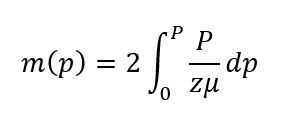If we assume a constant Z-factor and viscosity, the equation for pseudopressure simplifies to: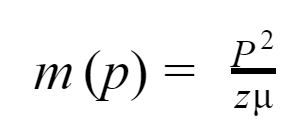Thus, the y-axis becomes:Where Pi is the initial reservoir pressure.

We use the square root of material balance time on the x-axis. Material balance time normalizes the plot for shut-in and changing rate/pressure conditions. This is necessary because the fundamental equations of RTA are derived for constant rate or constant pressure production. But in real data, bottomhole pressure and production rate change over time. Material balance time is equal to the cumulative production at time n, divided by the production rate at time n (Jha et. al., 2017). For constant rate production, material balance time equals time.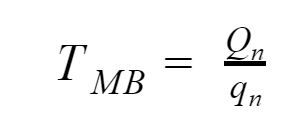For example, in the figures below, we can see that by plotting dP/q versus the square root of material balance time we get a nearly straight line. Plotting the same dataset versus the square root of time results in the dP/q trend deviating from straight line behavior significantly.Time does not necessarily increase monotonically from left to right when using material balance time. If q(n+1) is greater than q(n) by a sufficient margin, then T_MB(n+1) < T_MB(n). While this is unlikely to occur during uninterrupted flow, there are particular times when this phenomena is seen: during initial clean-up, installation of artificial lift, and/or coming on stream after a long shut-in.

In the example below, the well produces at a constant 1000 psi BHP for 5000 hours, then the BHP is abruptly changed to 500 psi. As a result, the production rate, q, increases and material balance time becomes much smaller; thus, the top line is the original production at 1000 psi and the bottom line corresponds to production at 500 psi.Jha et. al. (2017) discusses some of the shortcomings of material balance time. They identified that under certain conditions of fracture conductivity damage, linear flow may be identified later when using square-root material balance time versus using square-root time. However, they also found that under circumstances without damage, material balance time may result in more accurate identification of linear flow. For the purposes of assisting with history matching a ResFrac simulation and identifying physical processes present, material balance time is sufficiently accurate and mitigates uncertainties introduced by non-constant rate production.

Motifs

To develop motifs that can be used for diagnosing reservoir behavior, we run ResFrac simulations under a variety of conditions. Then, we construct RTA plots and look for signatures that can be used to distinguish different phenomena. Several SPE papers have been written that document the impact of various phenomena on RTA, and we use those as inspiration when compiling a list of testable scenarios (Jackson et. al., 2012). Comparison with published results provides validation.

To investigate, we construct a model with a single, pre-existing fracture. As we are testing the impact of individual variables on the RTA signature, we choose to use the pre-existing fracture to eliminate the complications introduced by injection and initial flowback on the RTA plot. We also initialize the model as 100% water saturated to mitigate any convoluting signatures from multi-phase effects.

We test the following variables for impact on RTA:

• Matrix (or “System”) Permeability

• Fracture Conductivity

• Distance to Reservoir ‘Boundaries’

• Pressure Dependent Permeability

• GOR and Bubble Point

Base Model

The base model is a single-phase, 100% water saturated reservoir with a single pre-existing fracture. Permeability is 50 nd in the horizontal direction, and vertical permeability is zero.

The pre-existing fracture is a single, rectangular fracture with a total length of just under 2000 feet and 150 feet in height. Because the hydraulic fracturing process is not modeled in the simulation, proppant transport is not modeled, and the fracture cannot be modeled as a propped hydraulic fracture. Instead, the aperture and conductivity of the pre-existing fracture is specified as part of the model setup.Impact of Permeability on RTA

We ran simulations with three different permeability values. The base case has a permeability of 50 nd and an effectively infinite conductivity fracture. Global permeability multipliers of 10 and 0.1 were applied in the K10 and K01 cases, respectively. As detailed in the literature (Belyadi et. al., 2015) higher permeability is associated with a shallower slope in the RTA plot.The slope of the line in the RTA plot is proportional to A*k^(1/2). If we take the slope of the base case (green line below) and that of the high permeability case (red line below), we calculate slopes of 37 psi-days/STB-day^(1/2) and 120 psi-days/STB-day^(1/2), respectively. Taking the ratio of the slopes we calculate a A*k^(1/2) ratio of 3.24. As the fracture areas are the same in the two cases, area cancels, and a permeability (k) ratio of 10 is calculated by squaring 3.24, matching the model inputs.Impact of Fracture Conductivity

Using the base model again, the impact of fracture conductivity was assessed by modifying the aperture of the pre-existing fracture. The aperture is decreased by a factor of 10 and 100 in the E001 and E0001 simulations. Conductivity is calculated as the cube of aperture, and so these simulations represent a 1000x and 1,000,000x decrease in conductivity from the base-case simulation. In the RTA plot, we see that values of reduced fracture conductivity correspond to progressively larger y-intercepts, as also shown in Jackson et. al., 2012.In the plot above, the base case with effectively infinite acting conductivity has a y-intercept of 0, as expected. As conductivity is reduced in the E001 and E0001 cases, the final slope of the RTA trend remains constant (as system permeability is equal in all cases), but the y-intercept increases:With progressively lower fracture conductivity, the system takes longer to reach linear flow (straight-line RTA behavior when plotted against square root time, or material balance time, on the x axis), and in the E0001 case, the occurrence of linear flow is just beginning. Prior to linear flow, we can see bi-linear flow as a straight line on a plot of dP/q versus the quarter root of material balance time, as below:In the image below, the simulation with E0 = 0.0001 was ran for a longer period of time so that linear flow could more fully be realized.Linear flow regimes are also apparent when viewing the simulation results directly. In the image below, we see the pressure distribution in the fracture at 10,000 hours. In the case of infinite-acting fracture conductivity, the entire fracture is drawn down to the BHP. In the cases with finite conductivity, we see a pressure gradient within the fracture, the degree of which scales with the E0 value.Impact of Reservoir Boundaries

Flow boundaries cause a signature in RTA plots. A flow boundary may be a sealing fault or another well, but in a multi-stage horizontal well would most commonly be interpreted as offsetting fractures initiating from adjacent clusters. The image below shows the pressure depletion from model with 10nD permeability. We observe that due to the low permeability, the region of pressure depletion around the fracture is quite narrow.We confirm this using the radius of investigation concept.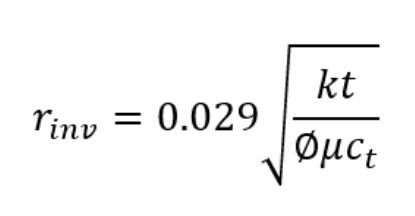Plugging in the parameters for this simulation (k = 1e-05, t = 10,000hrs, phi = 0.07, mu = 0.45cp, ct = 1.2e-05) we get a radius of 15ft. By increasing permeability by 10x, the radius of investigation increases by approximately three times. Thus to investigate the impact of boundary effects on RTA, we chose to increase the permeability of the simulation model by 10x such that the radius of investigation at 10,000 hours is 47 feet.In the image above, we observe the pressure depletion at 10,000 hours with 10x global permeability multiplier is much greater than in the prior simulation case.

Using this as the base case model (400ft matrix length along wellbore strike), we investigate shrinking the mesh in the x-direction (x-axis is oriented with the wellbore) to 1/8th and 1/16th of the original length, or 50ft and 25ft respectively. This puts a no-flow boundary 25 and 12.5 feet from the fracture for the two cases, well within the 47 foot radius of investigation. From the RTA plot, we observe that in both cases the depletion reaches the bounds of the reservoir as indicated by the upward curvature of the trend.Clear deviation from straight-line behavior occurs at approximately 23 and 13 square root material balance time for the case of 1/8th and 1/16th boundary cases, respectively. These times correspond to 4879 and 1500 hours in the respective simulations. The figure below shows the pressure depletion in both cases. Unlike the base case, pressure depletion reaches the mesh boundaries.Pressure Dependent Permeability (PDP)

The RTA motif of boundary effects is the upward curvature of the dP/q trend. As we know, the slope of the RTA trend is related to the permeability of the formation, and that lower permeabilities are associated with steeper RTA trends. If permeability is reduced during depletion, would we see an upward curve in the RTA trend?

Pressure dependent permeability has been documented in several unconventional basins. For instance, a 2013 paper by Heller et. al., ‘Experimental investigation of matrix permeability in gas shales’ shows up to an order of magnitude permeability reduction in Eagle Ford shale samples (Heller, et. al. 2013). If ‘system’ permeability is dependent on unpropped fracture conductivity (either natural or induced), then system may be even more pressure dependent.

In ResFrac we can specify either reversible or irreversible permeability multipliers. To test the effect on the RTA trend, we create three simulations:

• noPDP: no pressure dependence

• PDP: permeability reduction of 50% every 1000 psi

• PDP_max: permeability reduction of 75% every 1000 psi

The chart below shows the permeability multiplier in each of these cases.In the ResFrac visualization tool we can visualize the PDP process. The image below shows several snapshots through time for the PDP simulation. As the region of pressure depletion grows, so does the region of permeability alteration and the larger the pressure depletion, the larger the permeability change.In all simulations, initial reservoir pressure is 3500 psi, and the well is operated at a bottom hole pressure of 1000 psi, for a maximum delta pressure of 2500 psi. Additionally, the initial permeability in all cases is 10 nD. In the noPDP case, permeability remains constant throughout the duration of the simulation, and as such we should expect straight-line RTA behavior (matrix boundaries are set sufficiently far from the fracture). In the PDP and PDP_max scenarios, permeability reduces as a function pressure depletion, and thus we would expect to see a steepening of the RTA trend through time. The RTA plot of the three scenarios is shown below:The plot demonstrates the impact of PDP and the relative impacts of the different multipliers. The noPDP case exhibits straight-line behavior while PDP and PDP_max display steepening slopes. Note that the RTA trends in all three scenarios show the same initial slopes, indicative of equal initial permeabilities. The slopes of the RTA trends also correlate inversely to the permeability multipliers, with the PDP_max trend showing a steeper slope than the PDP case.

Similar to the boundary effect, we see that PDP can be another possible explanation for an upward curvature in the RTA trend. We might expect that in some instances both PDP and boundary effects will be present. With both effects present the upward curvature should be exacerbated. To test, we use the same three cases as above, but shrink the matrix region from 400ft to 10ft. Using the radius of investigation concept again, we should see the boundary at approximately 1200 hours or 10 square-root material balance hours in the base (noPDP) case.The resulting RTA trends confirm our expectations. The noPDP_bound scenario deviates from linear at 10 square-root material balance hours. At the same point, we see the upward curvature of the PDP_bound and PDP_max_bound trends exaggerated, evidence that the boundary is being “felt” while the additional effect of the PDP accounts of the progressively steeper slopes in the PDP_bound and PDP_max_bound cases.

Relative Permeability Effects

So far we have discussed only single-phase effects. Multiphase effects could fill an entire blog post separately. However, we will investigate one multiphase effect before concluding. In the sections above we showed that either boundary dominated flow or PDP can create an upward bending RTA trend. PDP results in the upward curvature due to the progressive degradation in permeability. How is an RTA curve affected when pressure drops below the bubble point (when the effective permeabilities of the liquid phases are reduced)?

To investigate, we perform two simulations with 100% oil saturation and initial solution gas-oil ratio of 2400 SCF/STB. In the ‘undersat’ scenario plotted below, the bubble point is set to 1000 psi (initial reservoir pressure is 6000 psi). In the ‘saturated’ scenario, bubble point is set to 4600 psi.Both simulation scenarios exhibit a linear slope until approximately a square-root material balance time of 5. At this point, the undersat scenario wiggles a little (numerical artifact), but the trend remains linear until the end of the simulation. In the saturated scenario, the reservoir begins dropping below bubble point at a square-root material balance time of 5 (corresponding to simulation hour 300). At this point gas begins to evolve and the relative permeability of the oil is reduced. This causes the RTA plot to curve upward. The plot below shows the GOR trend for the two simulations.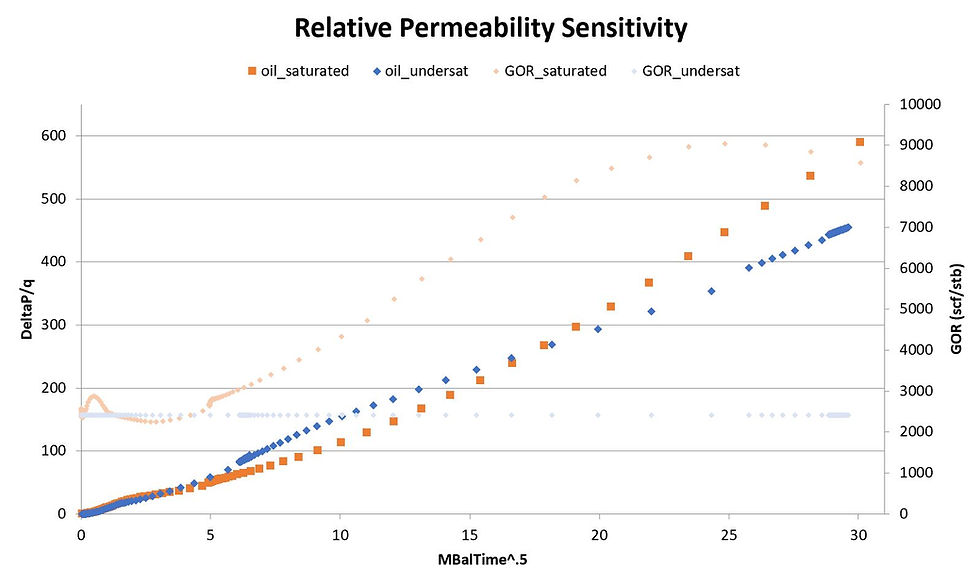In the ResFrac image below, I have displayed the production (upper left), pressure in the fracture (upper right), oil relative permeability in the matrix (bottom left), and the gas saturation (bottom right). Because the fracture has effectively infinite conductivity (aperture of ~0.002 inches, and no pressure gradient inside the fracture), the reservoir is being drawn down below bubble point all the way along the fracture, resulting in gas flow into the fracture along its entire length, and a commensurate reduction in the relative permeability of the oil in the matrix elements near the fracture as shown in the ResFrac screenshot below. As we saw in the RTA trend, this has the effect of reducing the effective permeability and causing the trend to curve upwards.Note that dropping below bubble point does not necessarily create an upward curvature in the RTA plot. The full equation for the slope of the RTA plot is below. The h*xf*sqrt(k) product has been the focus of our investigation so far. However, note that that ct is in the denominator. An increase in ct will result in a flattening of the trend when all other parameters are held equal. Going below bubble point will decrease oil relative permeability but increase the total compressibility. The net effect depends on the relative strength of these two processes.The plot below shows an example where dropping below bubble point results in an oil production increase. The oil used in this case was a heavier oil than in the previous case, as seen in the Rs of 800 scf/stb versus the 2400 scf/stb in the prior example.Summary

RTA is a simplified analysis technique and cannot capture many processes important in real data (vertical layering and heterogeneity, multiphase effects, complex fracture geometry, etc.). Nevertheless, it is a useful tool for diagnosing reservoir behavior and assisting history matching. The process is:

1. Build an RTA plot of the measured data

2. Run an initial simulation and build an RTA plot of the simulated data

3. Compare the simulations using the motifs presented above to help adjust simulation parameters:

4. If the field data exhibits a non-zero intercept, reduce fracture conductivity in the simulation to produce non-zero intercept

5. If the field data exhibits a straight-line slope steeper/shallower than the simulation, decrease/increase permeability in the simulation

6. If the field data exhibits upward curvature

7. What is cluster spacing and permeability? Is cluster spacing leading to boundary dominated flow?

8. Is the well/pad of interest in a basin that has documented PDP?

9. Does upward curvature correspond to a GOR increase? Could permeability reduction be due to relative permeability effects?

10. Iterate to refine history match

RTA provides an estimate for A*sqrt(k), but cannot independently estimate permeability and area. In our upcoming URTeC paper on DFIT interpretation, we discuss how DFITs can be used to estimate permeability, breaking the nonuniqueness from RTA analysis. We work through an example in which conventional DFIT interpretation yields a nearly 100x overestimate of permeability. When combined with RTA analysis, this would result in a nearly 10x underestimate of fracture length/area. At ATCE in the fall, we will have a paper discussing how inaccurate estimates of permeability and effective fracture length can profoundly impact well spacing, fracture design, and overall economics.

References

Belyadi, H., Yuyi, S., & Junca-Laplace, J.-P. (2015, October 13). Production Analysis Using Rate Transient Analysis. Society of Petroleum Engineers. doi:10.2118/177293-M

Heller, R., Vermylen, J., Zoback, M. (2014, May). Experimental investigation of matrix permeability of gas shales. AAPG Bulletin, v. 98, no. 5, pp.975-995.

Jackson, G., & Rai, R. R. (2012, January 1). The Impact Of Completion Related Pressure Losses On Productivity In Shale Gas Wells. Society of Petroleum Engineers. doi:10.2118/162213-MS

Jha, H. S., & Lee, W. J. (2017, July 24). Problems With Application of Material Balance Time to Transient Flow Data in Diagnostic Plots. Unconventional Resources Technology Conference. doi:10.15530/URTEC-2017-2697627